# AP Chemistry Practice Test 37

### Test Information12 questions18 minutes

1. Which of the reactions below will become thermodynamically favored only at high temperatures?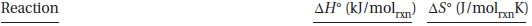`Questions 2-8 refer to the following information.Sodium azide, NaN3, is a component of automobile airbags. It is useful because it quickly decomposes to generate a large volume of nitrogen gas. The balanced chemical equation for the reaction is:2 NaN3(s) → 2 Na(s) + 3 N2(g)There are additional components in the airbag to react with the elemental sodium formed.Sodium azide is an unstable compound; therefore, it is often necessary to analyze samples as a check of its purity. A chemist is attempting to develop a new analytical, which employs the following reaction:NaN3(aq) + Na2S(aq) + 3 H2O(l) → N2(g) + NH3(g) + S(s) + 3 NaOH(aq)The chemist weighed a small flask both with and without a sample of sodium azide and recorded the masses. Next, she connected the flask to the system shown below.The flask in the middle and the rubber tubing leading to the beaker were completely filled with dilute acid, and then the clamp was removed. Excess sodium sulfide solution was added to the flask containing the sample. The liquid level in the second flask dropped as the generated nitrogen gas displaced the water into the beaker. The system was left intact until gas generation ceased. After the system returned to room temperature, the beaker was raised until the water in the beaker was at the same level as in the second flask. When the liquid levels were the same, the clamp was replaced to prevent further transfer. The chemist completed the following data table in her lab book.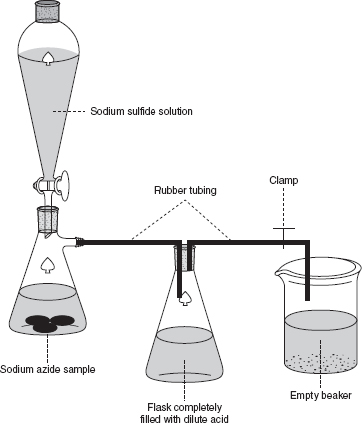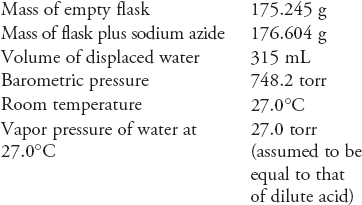`

2. What type of reaction generated the nitrogen gas?

3. Why must the liquid in the flask be dilute acid?

4. What is the partial pressure of the hydrogen gas in the flask?

5. After the system returned to room temperature an adjustment was made by raising the beaker until the liquid level in both the beaker and the flask were the same. Why was this step necessary?

6. Approximately, how many moles of nitrogen gas formed?

7. If the sample were pure sodium azide, approximately how many moles of nitrogen gas would form?

8. Would it be possible to use this experimental setup to study a reaction that produced gaseous sulfur dioxide, SO2? If not, why?

9.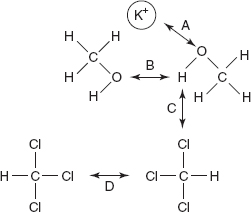Which of the labeled arrows in the diagram above represents the strongest intermolecular force of the four indicated?

10. If about 88% of a sample of pure 131I decays in 24 days, what is the approximate half-life of 131I?

11. Cycloalkanes are hydrocarbons with the general formula CnH2n, where n ≥ 3. If a 0.420 g sample of any alkene is combusted in excess oxygen, how many moles of water will form?

12.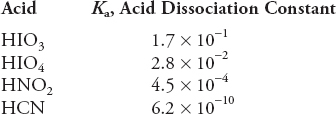A buffered solution with a pH near 5 is needed for an experiment. Using the above information, which of the combinations would be the best choice to prepare the buffer?